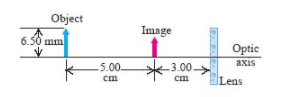# Problem: (Figure 1) shows an object and its image formed by a thin lensPart A) What is the focal length of the lens?Part B) What type of lens? (Converging or Diverging)Part C) What is the height of the image?Part D) Is it Real or Virtual?

###### FREE Expert Solution

Lens equation:
$\overline{)\frac{\mathbf{1}}{{\mathbit{s}}_{\mathbit{o}}}{\mathbf{+}}\frac{\mathbf{1}}{{\mathbit{s}}_{\mathbit{i}}}{\mathbf{=}}\frac{\mathbf{1}}{\mathbit{f}}}$

81% (107 ratings)###### Problem Details

(Figure 1) shows an object and its image formed by a thin lensPart A) What is the focal length of the lens?

Part B) What type of lens? (Converging or Diverging)

Part C) What is the height of the image?

Part D) Is it Real or Virtual?